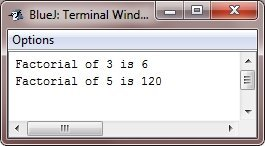# Java Recursion

Recursion is the process of defining something in terms of itself. As it relates to Java programming, recursion is the attribute that allows a method to call itself.

A method that can call itself is said to be a recursive method.

## Java Recursion Example

The classic example of recursion is computation of the factorial of a number. The factorial of a number say N is the produce of all the whole numbers between 1 and N. For example, the factorial of 3 is 1 * 2 * 3, or 6. Following is how a factorial can be computed by the use of recursive method:

```/* Java Program Example - Java Recursion */
/* This is a simple program of recursion */

class Factorial
{
/* this is a recursive method */
int fact(int n)
{
int result;

if(n == 1)
{
return 1;
}
result = fact(n-1) * n;
return result;
}
}

public class JavaProgram
{
public static void main(String args[])
{

Factorial f = new Factorial();

System.out.println("Factorial of 3 is " + f.fact(3));
System.out.println("Factorial of 5 is " + f.fact(5));

}
}```

When the above Java program is compile and executed, it will produce the following output:## Java Recursion Working Explanation

If you are unfamiliar with the recursive methods, then the operation of the fact() may seem a bit confusing. Here is how it works :
When the fact() is called with an argument of 1, the function returns 1; otherwise, it return the product of the fact(n-1)*n. To evaluate this expression, the fact() is called with n-1. This process repeats until n equals to 1 and the calls to the method begin returning.

To better understand how the fact() method works, let's go through a short example. When you compute the factorial of 3, the first call to the fact() will cause a second call to be made with an argument of 2. This invocation will cause the fact() to be called a third time with an argument of 1. This call will return 1, which is then multiplied by 2 (the value of n in the second invocation). This result (which is 2) is then returned to the original invocation of the fact() and multiplied by 3 (the original value of n). This yields the answer 6.

When a method calls itself, new local variables and parameters are allocated storage on the stack, and the method code is executed with these new variables from the start. As each recursive call returns, the old local variables and parameters are removed from the stack, and execution resumes at the point of call inside the method.

Recursive version of many routines may execute a bit more slowly than the iterative equivalent because of the added overhead of additional method calls. Many recursive calls to a method could cause a stack overrun. Because storage for the parameters and local variables is on the stack and each new call creates a new copy of these variables, it is possible that the stack could be exhausted. If this occurs, the Java run-time system will cause an exception. However, you probably will not have to worry about this unless a recursive routine runs wild.

The main advantage of recursive methods is that they can be used to create clearer and simpler version of several algorithms than can their iterative relatives.

Important - When writing recursive methods, you must have an if statement somewhere to force the method to return without the recursive call being executed. If you don't do this, once you call the method, it will never return. This is a very common error in working with recursion.

Tools
Calculator